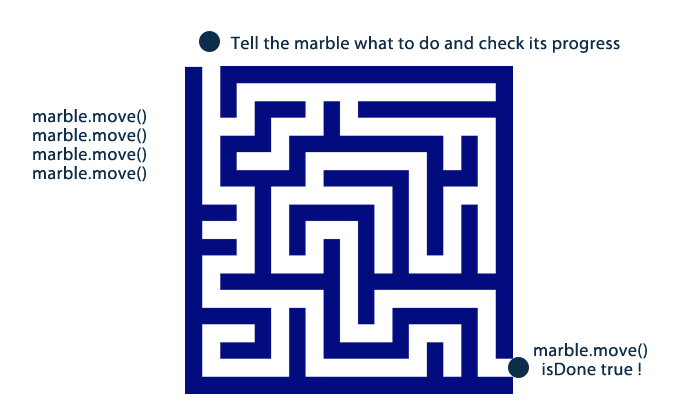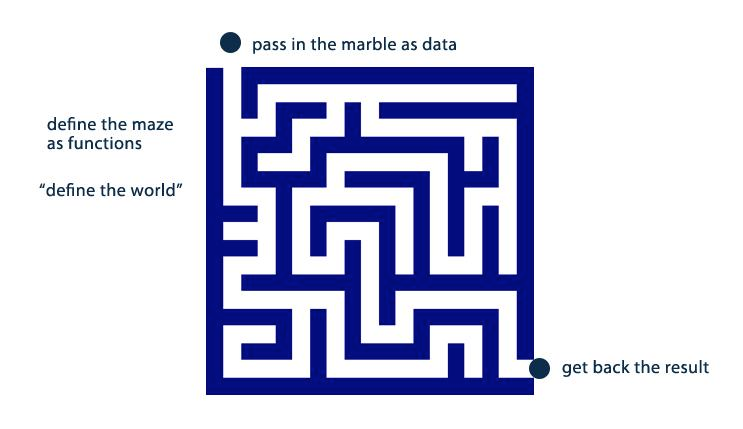# Functional JS

### IDEAS AND PRACTICE

• Imperative
• Declarative
• OOP - imperative, focuses on nouns
• Functional - declarative, focuses on verbs
• Logic, agent-oriented, component-based

## Imperative

• Todo list
• Fixed execution order
• HOW it should be done
• function is a subroutine (repeatable)## Declarative

• Expresses logic
• No fixed execution order
• expresses WHAT gets done
• defines "the world", data passes through## The State of OOP## Functional Programming

• Based in math
• Return an output based on input
• Functions are the PRIMARY abstractions vs. objects
• Iterate data structures and apply functions to return new structure
• Can still use objects
• OOP = collection of interacting, changing objects
• FP = stateless function evaluations that can be sequenced in various ways

## Purity

A "pure" function is a function that takes an input and returns a value
This is also called "referentially transparent"

``````
var f = function(x) { return x + 1; }
f(3);
//=> 4
``````

f(3) will ALWAYS = 4

## Mutability

• Mutable: can change
• Immutable: cannot change, new version constructed

## Purity has it's regrets..

In basic math, when you define a function there is no notions of a persistent "world" outside of it

``f(x) = x + 1 #=> f(3) = 4``

functions have no state...

Whenever we modify program state or talk to something outside of a function this is called a Side Effect - and should be a first class consideration

## Functional Idioms• map
• reduce
• reduceRight
• filter
• forEach
• every
• some

## Map

Transform a collection into a new one

``````
var arr = [1,2,3];
for (var i=0; i < arr.length; i++) {
arr[i] += 1;
}
``````
``````

// Arrays (native js)
[1, 2, 3].map(function(num) { return num + 1 });
//=> [2, 3, 4]

// Objects (lodash)
_.map({ 'one': 1, 'two': 2, 'three': 3 }, function(num) { return num * 3; });
//=> [3, 6, 9]

``````

## Reduce

Reduce a collection into a single value
``````

var arr = [1,2,3];
var results = 0;
for (var i=0; i < arr.length; i++) {
results += arr[i];
}

``````
``````
// Array (native JS)

[1, 2, 3].reduce(function(memo, num) { return memo + num });
//=> 6

// Objects (lodash)

_.reduce({ 'a': 1, 'b': 2, 'c': 3 }, function(result, num, key) {
result[key] = num * 3;
return result;}, {});
//=> { 'a': 3, 'b': 6, 'c': 9 }

``````
``` ```

## Reduce Right

``````
var list = [[0, 1], [2, 3], [4, 5]];
list.reduceRight(function(a, b){ return a.concat(b); });
//=> [4, 5, 2, 3, 0, 1]
``````

## Filter

Filter items out of an array

``````
var arr = [1,2,3,4,5];
var evens = []
for (var i=0; i<arr.length; i++) {
if (arr[i] & 2) {
evens.push(arr[i]);
}
}
``````
``````
// get evens
var arr = [1,2,3,4,5];
arr.filter(function(num){ return (num % 2 == 0) });
``````

## Foreach

Iterate each item

``````

var arr = [1, 2, 3];

arr.forEach(function(x){
// do something outside of me
console.log(x);
});

``````

## Every

Something must be true for every item

``````
var isOdd = true;
var arr = [1,2,5,7,9];
for (var i=0; i<arr.length; i++) {
if ((arr[i] & 1) === 0) {
isOdd = false;
break;
}
}
``````
``````
[1, 2, 3, 4, 5].every(function(item){ return item >= 3; });
//=> false
``````
``` ```

## Some

Function is true for some items

``````
var hasOdd = false;
var arr = [1,2,5,7,9];
for (var i=0; i<arr.length; i++) {
if ((arr[i] & 1) === 1) {
hasOdd = true
break;
}
}
``````
``````
[1, 2, 3, 4].some(function(item){
return item >= 3;
});
``````

## Lodash

Has everything you could want

## Compose

Combine 2 functions

f1(f2())

``````
var hello = function(name){ return "hi" + name; }
var place = function(place){ return place + "!"; }
var hi = _.compose(hello, place);
hi("world");
//=> hi world!
``````

## Bind/Curry

http://repl.it/Mv3/5

``````
var book = function(name) {
console.log(name);
}

angular = book.bind(null, "AngularJS In Action");
angular();

``````

## Bind with scope

``````

var book = function(review) {
console.log(this.name+" is "+review);
}

var mybook = book.bind({name:'foo'}, "good!");
mybook();

``````

## Some code

``````
var flow = [

getUrls,
getDocs,
clearUnlessES,
findUrls,
filterDupes,
saveUrls

waterfall(flow, function(err) {

stats.end      = Date.now();
stats.duration = stats.end-stats.start;
cb(err, stats);

});

``````

By wearefractal

# Copy of Functional JS

Functional JS strategies

• 1,684